In this package you will find a series of functions for soil physics data analysis. These functions includes five models of water retention curve, seven methods of soil precompression stress, least limiting water range (LLWR), Integral Water Capacity (IWC), soil penetration resistance curve by Busscher's model, calculation of Soil Aggregate-Size Distribution, S Index, critical soil moisture and maximum bulk density using data from Proctor test, calculation of equivalent pore radius as a function of soil water tension, simulation of sedimentation time of soil particles through Stokes' law, simulation of soil pore size distribution, calculation of the hydraulic cut-off introduced by Dexter et al. (2008) and simulation of soil compaction induced by agricultural field traffic. Other utilities like functions to calculate the void ratio and to determine the maximum curvature point are available.

# Instalation

From CRAN:

``````install.packages("soilphysics")
``````

Or you can install the development version from GitHub:

``````install.packages("devtools")
devtools::install_github("arsilva87/soilphysics")
``````

``````library(soilphysics)
#> ---
#> soilphysics version 4.0
#>
``````

## Soil compaction tools

Using the function stressTraffic, it it possible calculate the contact area, stress distribuition and stress propagation based on the SoilFlex model.

``````stress <- stressTraffic(inflation.pressure=200,
recommended.pressure=200,
tyre.diameter=1.8,
tyre.width=0.4,
conc.factor=c(4,5,5,5,5,5),
layers=c(0.05,0.1,0.3,0.5,0.7,1),
plot.contact.area = TRUE)
``````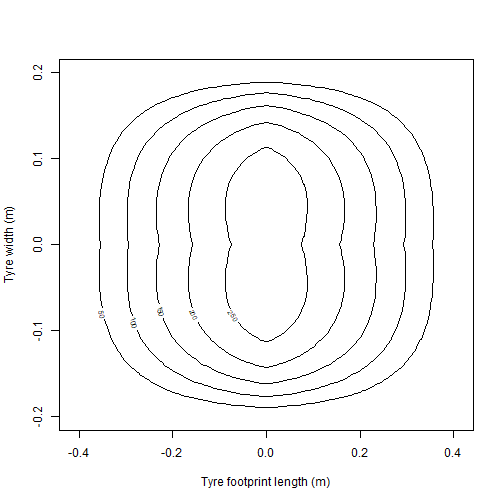Unsing the funtion soilDeformation, it is possible calculates the bulk density variation as a function of the applied mean normal stress using critical state theory, by O'Sullivan and Robertson (1996).

``````soilDeformation(stress = 300,
p.density = 2.67,
iBD = 1.55,
N = 1.9392,
CI = 0.06037,
k = 0.00608,
k2 = 0.01916,
m = 1.3,graph=TRUE,ylim=c(1.4,2.0))
``````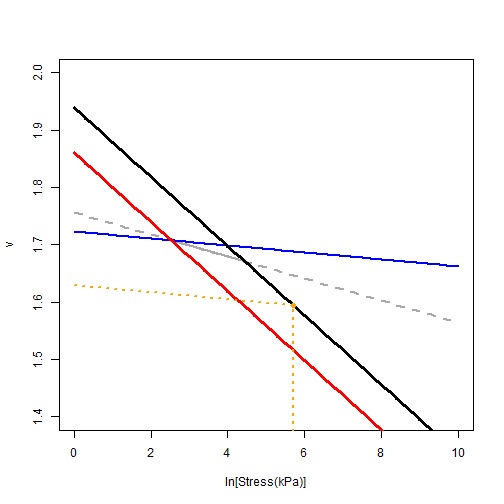``````#>    iBD    fBD     vi     vf   I%
#> 1 1.55 1.6385 1.7226 1.6295 5.71
``````

## Critical soil moisture and maximum bulk density (Proctor test)

``````mois <- c(0.083, 0.092, 0.108, 0.126, 0.135)
bulk <- c(1.86, 1.92, 1.95, 1.90, 1.87)
criticalmoisture(theta = mois, Bd = bulk)
``````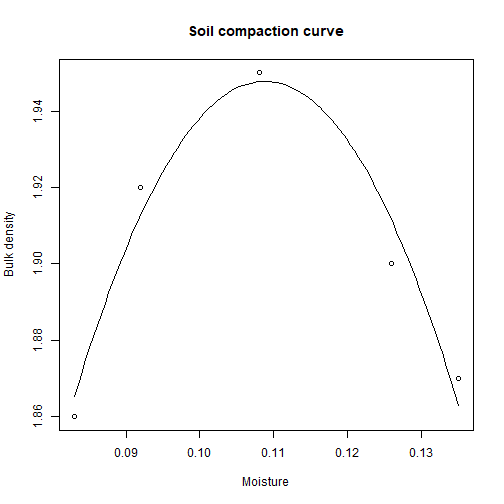``````#>
#>           Critical Moisture and Maximum Bulk Density
#>
#>                   Sample 1
#> Intercept        0.4825950
#> mois            26.9265767
#> mois^2        -123.7120431
#> R.squared        0.9515476
#> n                5.0000000
#> critical.mois    0.1088276
#> max.bulk         1.9477727
``````

## Soil water availability tools

Quantifying the soil water availability for plants through the IWC approach:

``````iwc(theta_R = 0.166, theta_S = 0.569, alpha = 0.029, n = 1.308,
a = 0.203, b = 0.256, hos = 200, graph = TRUE)
``````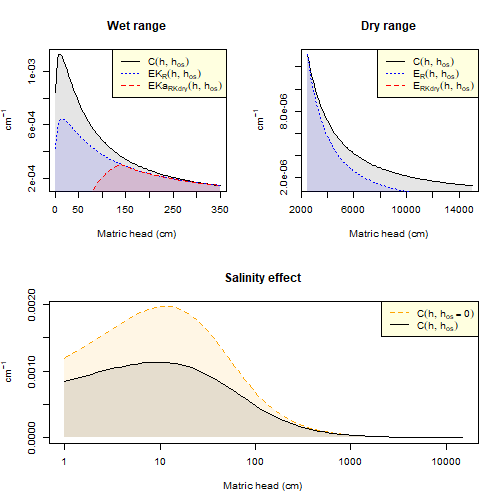``````#>                      IWC       EI         h.Range
#> EKa(h, hos)    0.0144000   0.9600  66.43 - 139.49
#> EK(h, hos)     0.0405000   5.3700    139.49 - 330
#> C(h, hos)      0.0846000  49.4800   330 - 2471.44
#> ER(h, hos)     0.0288000  87.1200 2471.44 - 15000
#> ERKdry(h, hos) 0.0006000   4.9100   12000 - 15000
#> Sum            0.1689139 147.8336       0 - 15000
``````

Quantifying the soil water availability for plants through the LLWR approach:

``````# Usage
data(skp1994)
with(skp1994,
llwr(theta = W, h = h, Bd = BD, Pr = PR,
particle.density = 2.65, air = 0.1,
critical.PR = 2, h.FC = 100, h.WP = 15000))
#>
#>           Least Limiting Water Range
#>
#> ----------
#> Limiting theta (6 first rows):
#>         thetaA   thetaPR   thetaFC   thetaWP
#> [1,] 0.3905660 0.2461742 0.3900669 0.2568751
#> [2,] 0.3226415 0.3104104 0.3694538 0.2433005
#> [3,] 0.3867925 0.2495628 0.3888922 0.2561015
#> [4,] 0.3490566 0.2846168 0.3773373 0.2484921
#> [5,] 0.3226415 0.3104104 0.3694538 0.2433005
#> [6,] 0.3566038 0.2774366 0.3796205 0.2499957
#>
#> ----------
#> Estimates of the soil water content model:
#>
#> Formula: theta ~ exp(a + b * Bd) * h^c
#>
#> Parameters:
#>    Estimate Std. Error t value Pr(>|t|)
#> a -0.150316   0.147075  -1.022  0.31080
#> b -0.301627   0.099303  -3.037  0.00351 **
#> c -0.083368   0.004142 -20.128  < 2e-16 ***
#> ---
#> Signif. codes:  0 '***' 0.001 '**' 0.01 '*' 0.05 '.' 0.1 ' ' 1
#>
#> Residual standard error: 0.02239 on 61 degrees of freedom
#>
#> Number of iterations to convergence: 3
#> Achieved convergence tolerance: 3.089e-07
#>
#> pseudo R-squared: 0.8855893
#>
#> ----------
#> Estimates of the soil penetration resistance model:
#>
#> Formula: Pr ~ b0 * (theta^b1) * (Bd^b2)
#>
#> Parameters:
#>    Estimate Std. Error t value Pr(>|t|)
#> b0  0.07684    0.02537   3.029 0.003598 **
#> b1 -1.66486    0.18007  -9.246 3.28e-13 ***
#> b2  3.08404    0.77814   3.963 0.000196 ***
#> ---
#> Signif. codes:  0 '***' 0.001 '**' 0.01 '*' 0.05 '.' 0.1 ' ' 1
#>
#> Residual standard error: 0.6293 on 61 degrees of freedom
#>
#> Number of iterations to convergence: 8
#> Achieved convergence tolerance: 4.84e-06
#>
#> pseudo R-squared: 0.6593648
#>
#> ----------
``````

Quantifying the LLWR using van Genuchten's parameters:

``````par(mfrow=c(1,2))
llwr_llmpr(thetaR=0.1180, thetaS=0.36, alpha=0.133, n=1.30,
d=0.005, e=-2.93, f=3.54, PD=2.65,
critical.PR=4, h.FC=100, h.PWP=15000, air.porosity=0.1,
labels=c("AFP", "FC","PWP", "PR"),
graph1=TRUE,graph2=FALSE, ylab=expression(LLMPR~(hPa)), ylim=c(15000,1))
#> \$CRITICAL_LIMITS
#>      theta potential
#> AIR 0.2600     41.02
#> FC  0.2285    100.00
#> PWP 0.1428  15000.00
#> PR  0.1939    356.65
#>
#> \$LLRW_LLMPR
#>          Upper    Lower    Range
#> LLWR    0.2285   0.1939   0.0346
#> LLMPR 100.0000 356.6500 256.6500
mtext(expression("Bulk density"~(Mg~m^-3)),1,line=2.2, cex=0.8)

llwr_llmpr(thetaR=0.1180, thetaS=0.36, alpha=0.133, n=1.30,
d=0.005, e=-2.93, f=3.54, PD=2.65,
critical.PR=4, h.FC=100, h.PWP=15000, air.porosity=0.1,
labels=c("AFP", "FC","PWP", "PR"),
graph1=FALSE,graph2=TRUE, ylab=expression(LLMPR~(hPa)), ylim=c(0.1,0.5))
#> \$CRITICAL_LIMITS
#>      theta potential
#> AIR 0.2600     41.02
#> FC  0.2285    100.00
#> PWP 0.1428  15000.00
#> PR  0.1939    356.65
#>
#> \$LLRW_LLMPR
#>          Upper    Lower    Range
#> LLWR    0.2285   0.1939   0.0346
#> LLMPR 100.0000 356.6500 256.6500
mtext(expression("Bulk density"~(Mg~m^-3)),1,line=2.2, cex=0.8)
``````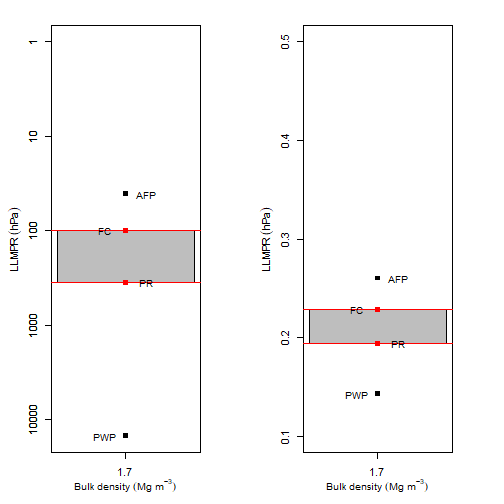## Precompression stress

Estimating the precompression stress by several methods:

``````pres <- c(1, 12.5, 25, 50, 100, 200, 400, 800, 1600)
VR <- c(0.846, 0.829, 0.820, 0.802, 0.767, 0.717, 0.660, 0.595, 0.532)
sigmaP(VR, pres, method = "casagrande", n4VCL = 2)
``````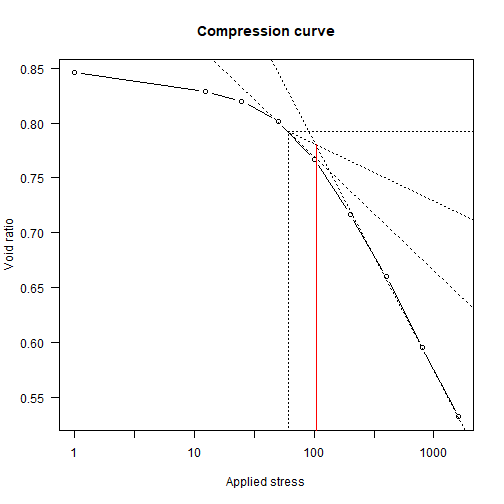``````#> Preconsolidation stress: 104.2536
#> Method: casagrande, with mcp equal to 1.7885
#> Compression index: 0.2093
#> Swelling index: 0.0179
``````

## Soil water retention curve

Fitting (interactive!) water retention curve using van Genuchten's model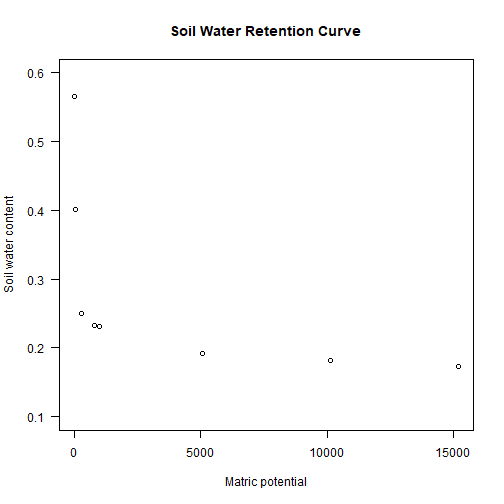## S Index

``````Sindex(theta_R=0, theta_S=0.395, alpha=0.0217, n=1.103, xlim = c(0, 1000))
``````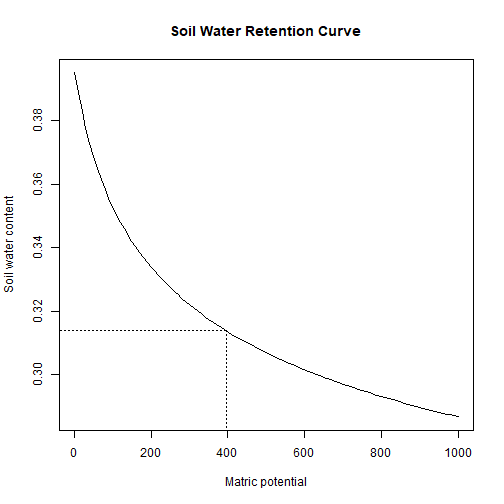``````#>
#>           The S Index
#>
#> h_i : 395.4757
#> theta_i : 0.3139
#> |S| : 0.0296
#> Soil physical quality : Poor
``````

## Soil Aggregate-Size Distribution

``````data(SoilAggregate)
#>   ID    D3 D1.5 D0.75 D0.375 D0.178 D0.053
#> 1 A1 25.80 7.55  5.50   5.10   3.00   3.05
#> 2 A2 19.85 5.30  7.45   7.30   4.40   5.70
#> 3 A3  7.10 9.80 11.60   8.10   2.35  11.05
#> 4 B1  6.10 4.85 11.20  13.10   7.15   7.60
#> 5 B2 12.00 6.30 16.10   7.35   3.70   4.55
#> 6 B3 14.10 6.15  8.80  11.05   4.60   5.30
classes <- c(3, 1.5, 0.75, 0.375, 0.178, 0.053)
out <- aggreg.stability(sample.id = SoilAggregate[ ,1],
dm.classes = classes,
aggre.mass = SoilAggregate[ ,-1])
#>   [sample.id] [MWD (mm)] [GMD (mm)] [Total mass (g)]  3 1.5 0.75 0.375
#> 1          A1   1.909163  1.2382214               50 52  15   11    10
#> 2          A2   1.538206  0.8239103               50 40  11   15    15
#> 3          A3   0.974829  0.4865272               50 14  20   23    16
#> 4          B1   0.811260  0.4311214               50 12  10   22    26
#> 5          B2   1.223620  0.7282644               50 24  13   32    15
#> 6          B3   1.267369  0.6853162               50 28  12   18    22
#>   0.178 0.053
#> 1     6     6
#> 2     9    11
#> 3     5    22
#> 4    14    15
#> 5     7     9
#> 6     9    11
``````

# Citation and references

De Lima, R.P.; Da Silva, A.R.; Da Silva, A.P. (2021) soilphysics: An R package for simulation of soil compaction induced by agricultural field traffic. SOIL and TILLAGE RESEARCH, 206: 104824. DOI: https://doi.org/10.1016/j.still.2020.104824

De Lima, R.P.; Tormena, C.A.; Figueiredo, G.C; Da Silva, A.R.; Rolim, M.M. (2020) Least limiting water and matric potential ranges of agricultural soils with calculated physical restriction thresholds. Agricultural Water Management, 240: 106299. DOI: https://doi.org/10.1016/j.agwat.2020.106299

Da Silva, A.R.; De Lima, R.P. (2017) Determination of maximum curvature point with the R package soilphysics. International Journal of Current Research, 9: 45241-45245.

De Lima, R.P.; Da Silva, A.R.; Da Silva, A.P.; Leao, T.P.; Mosaddeghi, M.R. (2016) soilphysics: an R package for calculating soil water availability to plants by different soil physical indices. Computers and Eletronics in Agriculture, 120: 63-71. DOI: https://doi.org/10.1016/j.compag.2015.11.003

Da Silva, A.R.; De Lima, R.P. (2015) soilphysics: an R package to determine soil preconsolidation pressure. Computers and Geosciences, 84: 54-60. DOI: https://doi.org/10.1016/j.cageo.2015.08.008

# Contributions and bug reports

soilphysics is an ongoing project. Then, contributions are very welcome. If you have a question or have found a bug, please open anor reach out directly by e-mail: anderson.silva@ifgoiano.edu.br or .